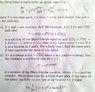# Using Partial Derivatives to check B-S Equation holds and find constants

• robot1000

#### robot1000

The question I'm trying to solve is part (ii) of the attached file

I've used partial derivatives to input back into the Black Scholes equations and after factorising it, I've got it down to:

(a + 2bt + αt +r) * (S².c.e^(at+bt²) = 0

I'm now stuck on what to do next, as there would need to be 2 equations in order to solve simultaneously which isn't the case above.

Help would appreciated

#### Attachments

•p1254191011.jpg
66.4 KB · Views: 452
I think I got the solution to the first part by letting (a + 2bt + αt +r) = 0

Therefore a = -r and b = -α/2

However I'm not sure how to complete the last part, I'm sure it's quite straight forward, but I just can't see what to do.

Thanks